Next: r-Algebras Up: Braids and Quantization Previous: The Quantum Plane

# r-Commutative Geometry

While there are plenty of things to say about the quantum plane, and quantum groups, I think I'll home in on my main topic at last: r-commutative geometry. This is a particular approach to noncommutative geometry that generalizes what mathematicians and physicists call supergeometry''. So first I should say a brief word about supergeometry. First, recall that the plain old geometry of manifolds can be cast into the language of commutative algebra by considering not the manifold itself as a set of points, but the algebra of (smooth, complex) functions on the manifold. (In this language, for example, vector fields are derivations, vector bundles are projective modules, and so on -- every geometric construct has an algebraic analog.) Now in the 1970's, physicists really caught on to the fact that considering only commutative algebras was horribly unfair to fermions, which like to anticommute: i.e. they have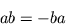So while the phase space of bosonic system is a manifold, the phase space of a system containing both bosonic and fermionic degrees of freedom is a supermanifold'' -- a (particular kind of) algebra which has even'' and odd'' elements, such that the even, or bosonic, elements commute, while the odd elements anticommute. (The even elements commute with the odd elements, by the way.) Generalizing lots of concepts from commutative algebras to supercommutative algebras simply amounts to sticking in appropriate minus signs! The rule of thumb is that whenever one switches two elementsand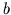, one should stick in a factor of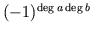, where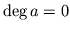ifis even (bosonic) and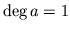ifis odd (fermionic). One may extend the notions of vector field, differential form, metric, curvature, and all your favorite concepts from geometry to supergeometry'' in this manner.

This turned out to be fascinating (it's a bit premature to say useful'') in particle physics, where it goes by the name of supersymmetry. The idea is that there should be a symmetry between bosons and fermions. While this is not at all observed in nature, it would be nice if it were true, so people have developed clever ways of rigging up their theories so that you never see the superpartners'' every particle has: for the photon, the photino, for the gluon, the gluino, for the leptons, schleptons, for the quarks, squarks... you get the pattern. (Don't complain to me if you think this is silly, it wasn't my idea!) Superstrings are the latest of these super'' ideas in particle physics.

Supersymmetry has actually proven itself in a more practical manner in nuclear physics, where it lets one model resonances in nuclei, relating the properties of fermionic and bosonic nuclei.

Where supergeometry really shines, though, is in mathematics. For example, Ed Witten came up with beautiful proofs of the Atiyah-Singer index theorem and the positive mass theorem (a theorem about general relativity) using supergeometry. (As usual, he left it to others to make his arguments rigorous.)

While I personally don't think that bosons and fermions were created equal in the manner postulated by supersymmetry, I do favor an approach to physics which doesn't take bosons, or commuting variables, to be somehow superior to fermions, or anticommuting variables. This demands supergeometry. For example, a decent treatment of classical fermions requires a supermanifold for the phase space''.

My own personal twist (motivated by the work of many people on anyons, the braid group, quantum groups, etc.) is to try to take a look at what geometry would be like if one wanted to be fair to anyons as well as bosons and fermions. This is r-commutative geometry.''

Recall that given a vector space, an invertible linear transformation, the Yang-Baxter equations say that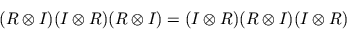whereis the identity on. The idea is thatswitches'' two elements of, mapping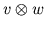to, and if we drawas a crossing,'' as follows:The Yang-Baxter equations say that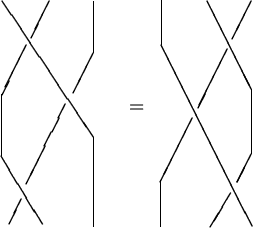Now suppose that our vector space is really an algebra -- let's call it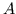. (I mean an associative algebra with unit.) The product in the algebra defines a multiplication map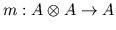, given by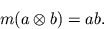We can draw this as the joining of two strands: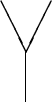Associativity simply says that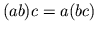, or in terms of diagrams,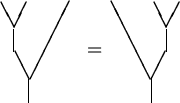Now these diagrams aren't really braids because of the fusion of strands'' that's taking place, but they fit in well with the braid group philosophy. For example, there is a sense in which the two trees I've drawn above are topologically the same, just as the Yang-Baxter equation expresses a topological identity. There are generalizations of braids, e.g. the ribbon graphs'' of Reshetikhin and Turaev, that make all this precise.

Now: an r-algebra'' is an algebraequipped with a solution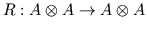of the Yang-Baxter equations such that:

1)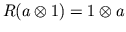and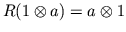for allin-- i.e., one switches'' the identity 1 within the usual manner;

2) the following conditions hold -- I'll draw them pictoriallly: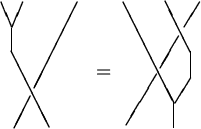and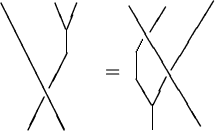If you like equations instead of pictures, these areand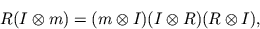as maps from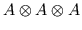to, respectively. The first one tells you how to compute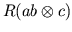, and the second one tells you how to compute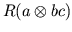, so both tell you how to switch a product of two elements past a third element. These are called the quasitriangularity'' conditions and are crucial in the theory of quantum groups (usually in a slightly disguised form).

Now commutativity says that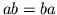-- in other words, you can multiply two elements of, or you can switch them first and then multiply them, and you'll get the same result. Generalizing this, we say that an r-algebra is r-commutative'' ifas maps fromto. In diagrams: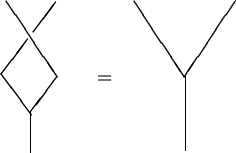In words: switch, then multiply, equals multiply.''

It turns out that one can do a fair amount of geometry for r-commutative algebras. And there are lots of examples of r-commutative algebras. In fact, many of the algebras obtained by quantization are r-commutative: for example, the Clifford algebra, the Weyl algebra, noncommutative tori, the quantum plane (and all other r-symmetric algebras), and quantum groups. Also, all supercommutative algebras are automatically r-commutative.

In fact, I believe that there is a deep relation between braids (or r-commutativity) and quantization. This goes back to Kauffman's observation that the skein relation for the Conway polynomial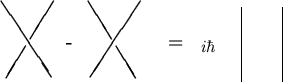and the canonical commutation relationsIt was made more clear by the discovery of the relation between quantum groups and knot invariants (which I haven't really touched upon yet - here I highly recommend Louis Kauffman's book Knots and Physics.) It was made still more clear (in my opinion) by the work of Frohlich, Gabbiani, Rehren, Schroer, and many others on the appearance of braid group statistics in low-dimensional quantum field theory. Essentially, these authors show that every nice quantum field theory gives rise to a fusion algebra'' for the conserved charges, and that these fusion algebras are r-algebras. But I suspect that at the root of it all is something rather simpler which we haven't understood yet. A clue, I think, lies in the classical Yang-Baxter equations.'' These are what physicists would call a semiclassical limit'' of the Yang-Baxter equations. Namely, takeof the form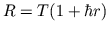, where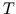is the usual twist map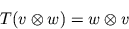and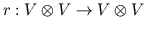, write down the Yang-Baxter equation for, and collect all terms of order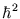. This equation is identical to the equation that a Poisson structure'' must satisfy. For details, see Drinfeld's famous review paper on quantum groups. The point here is that a Poisson structure, which defines the Poisson bracket of classical observables, is a semiclassical limit of the commutator which appears in quantum mechanics. Thus an infinitesimal deformation of the usual twist map that is required to satisfy the Yang-Baxter equation is the same thing as an infinitesimal deformation of the commutative product in the classical algebra of observables (i.e., a Poisson bracket).

More later...Next: r-Algebras Up: Braids and Quantization Previous: The Quantum Plane